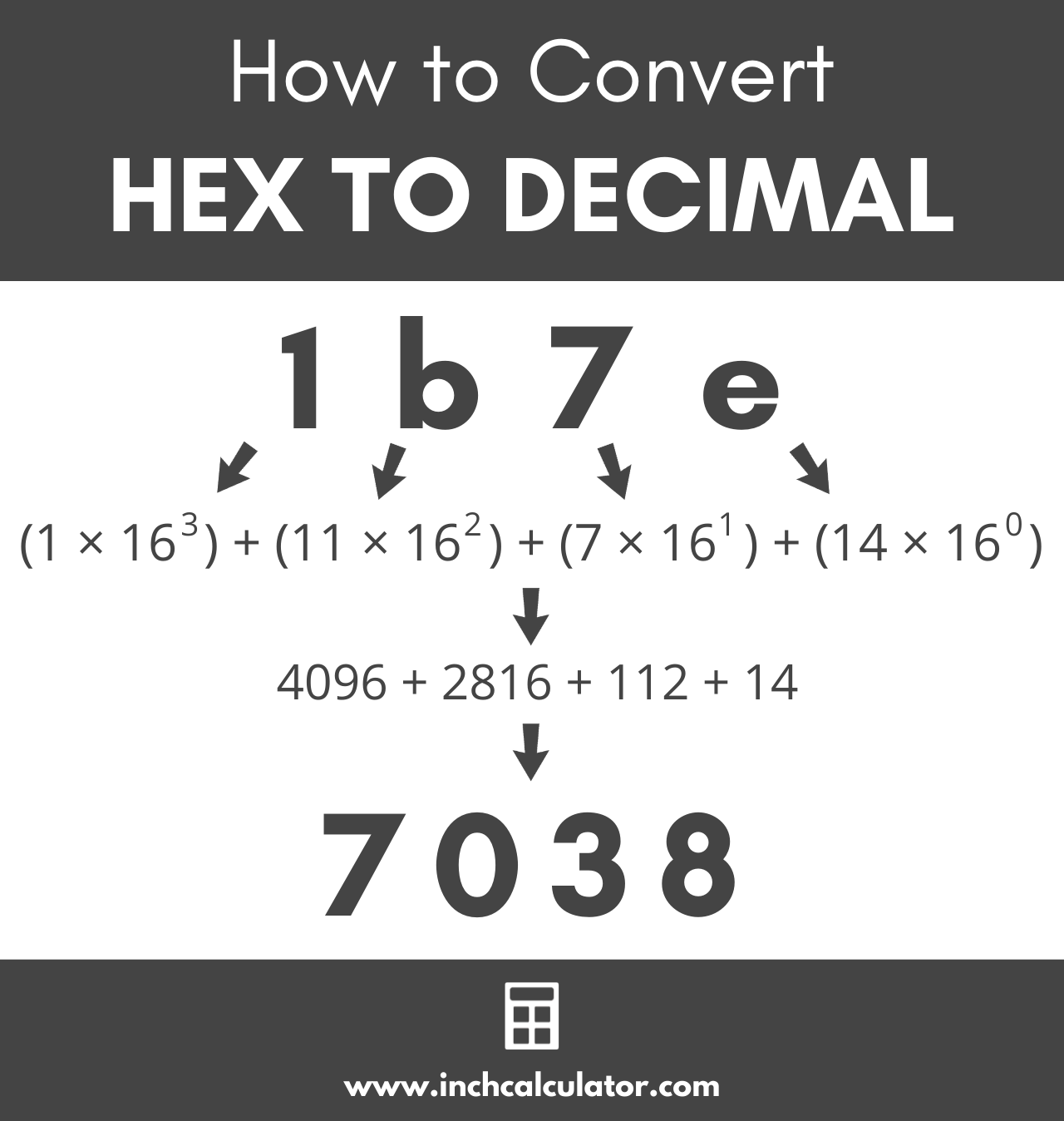Enter a hex number below to convert it to a decimal.

## Decimal Number:

### Steps to Convert to Decimal

Learn how we calculated this below

## How to Convert Hex to Decimal

The hexadecimal number system is a base 16 number system since it uses 16 digits 0 to 9 and a to f. On the other hand, decimal is a base 10 number system since it uses ten digits, 0 to 9.

Often you’ll need to convert a hex number to its decimal value since most people use the decimal system. Hexadecimal numbers are often used in software and networking applications.

To convert a hexadecimal number to a decimal, you can use the positional notation method. To use this method, multiply each digit in the hex number from the rightmost number to the left by 16 to the power of n, where n is the distance from the right.

So, reading the hex number from right to left, the furthest digit to the right is equal to the digit times 160. The digit that is one position from the right is equal to the digit times 161.

### Hex to Decimal Formula

Thus, the hex to decimal formula is:

decimal number10 = (d0 × 160) + (d1 × 161) + … + (dn – 1 × 16n – 1)

In this formula, d0 is the hex digit furthest to the right, d1 is the digit one position from the right, and dn – 1 is the digit furthest to the left.You can also use a tool like our hex calculator to convert to decimal or binary.

For example, let’s convert the hex number 1b7e to decimal.

decimal number10 = (1 × 163) + (11 × 162) + (7 × 161) + (14 × 160)
decimal number10 = 4096 + 2816 + 112 + 14
decimal number10 = 7038

## How to Convert Fractional Hexadecimal Numbers to Decimal

For real hex numbers that contain fractional values, you can use the method above to convert as well, with a slightly different exponent value. Start by using the method above to convert the whole portion of the number from hex to decimal.

Then, for the remaining fractional value, multiply each number to the right of the decimal point by 16 to the power of -1 times the distance from the decimal point plus 1. That might seem complicated but it’s easier than it sounds, let’s demonstrate.

decimal number10 = (d-1 × 16-1) + (d-2 × 16-2) + … + (d-n × 16-n)

For example, let’s convert the hex number 0.c3f to decimal.

decimal number10 = (12 × 16-1) + (3 × 16-2) + (15 × 16-3)
decimal number10 = (12 ÷ 161) + (3 ÷ 162) + (15 ÷ 163)
decimal number10 = 0.75 + 0.01171875 + 0.003662109375
decimal number10 = 0.765380859375

The calculator above can convert hex numbers to decimal, including fractional numbers like this one, along with negative numbers.

## Hex to Decimal Conversion Table

The table below shows hexadecimal numbers and the equivalent decimal number values.

Hexadecimal numbers converted to the equivalent decimal values
0 0
1 1
2 2
3 3
4 4
5 5
6 6
7 7
8 8
9 9
a 10
b 11
c 12
d 13
e 14
f 15
10 16
11 17
12 18
13 19
14 20
15 21
16 22
17 23
18 24
19 25
1a 26
1b 27
1c 28
1d 29
1e 30
1f 31
20 32
40 64
80 128
100 256
200 512
400 1024
800 2048

You might also be interested in our hex calculator to add or subtract hex numbers.